# DATEDIF Function

The DATEDIF function returns the number of years, months or days between two dates and is officially "unsupported".
In Excel 365 this function is still available for backwards compatibility but should not be used.
This function does not appear in the 'Insert Function' dialog box and is not recognised in the 'Function Arguments' dialog box.
The accuracy of this function was improved slightly in Excel 2010 but some of the bugs were never fixed.

### Known Bugs (M, MD and YD units)

There are known bugs when you use the "M" unit.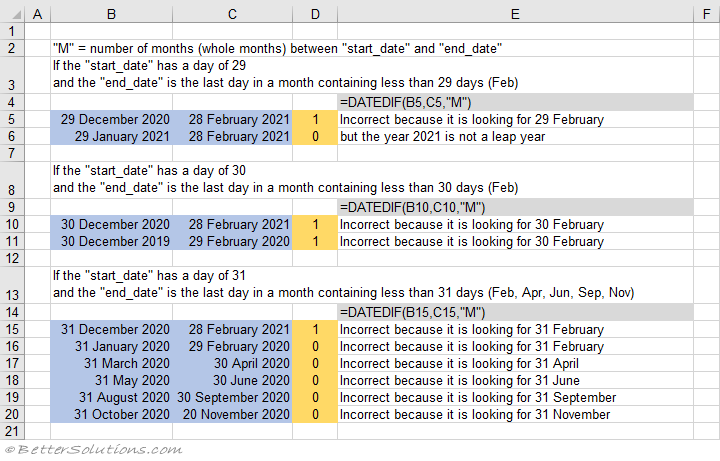There are known bugs when you use the "MD" unit.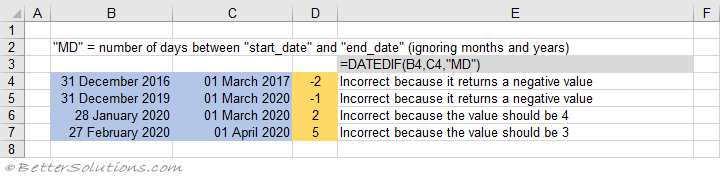There are known bugs when you use the "YD" unit.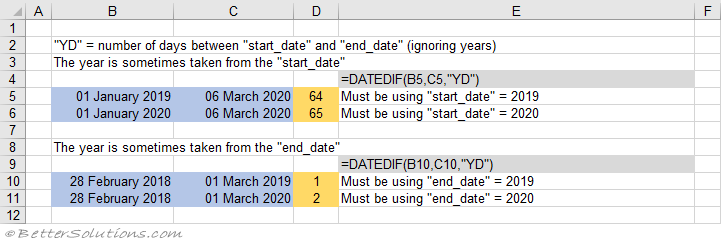### Replacement Formula - M

Column "D" uses the DATEDIF function and often gives the incorrect answer.
Column "E" uses the formula shown.
This formula uses the following functions: ROUNDDOWN, YEAR, MONTH, DAY, EOMONTH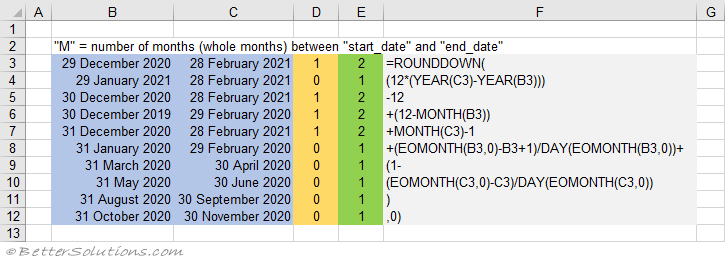### Replacement Formula - MD

Column "D" uses the DATEDIF function and often gives the incorrect answer.
Column "E" uses the formula shown.
This formula uses the following functions: DATE, YEAR, MONTH and DAY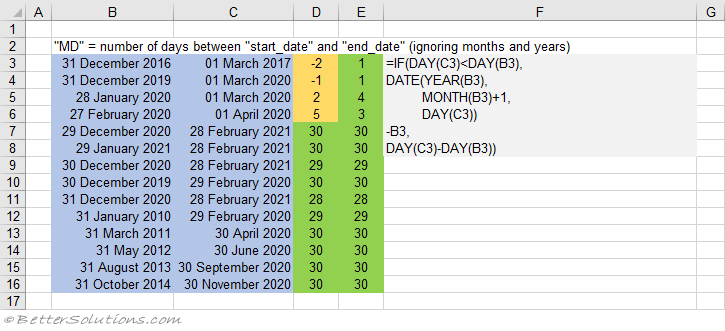### Replacement Formula - YD

Column "D" uses the DATEDIF function and gives the incorrect answer.
Column "E" uses the formula and gives the correct answer.
This formula uses the following functions: EDATE, ROUNDDOWN and YEARFRAC
This formula always takes the year from the "end_date".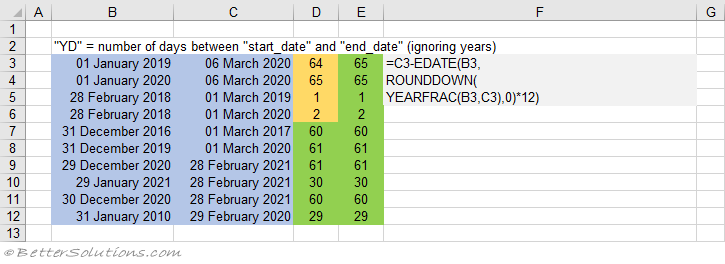### Replacement Formula - YM

Column "D" uses the DATEDIF function.
Column "E" uses the formula shown.
This formula uses the following functions: MOD, YEAR, MONTH and DAY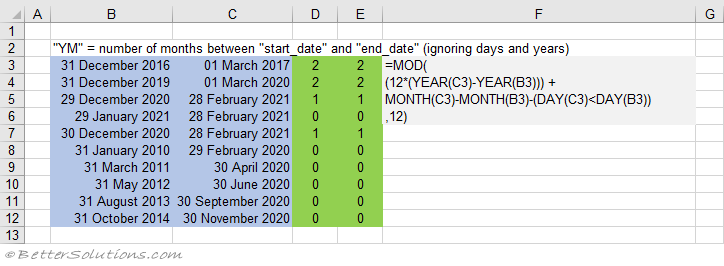### Replacement Formula - Y

Column "D" uses the DATEDIF function.
Column "E" uses the formula shown.
This formula uses the following functions: ROUNDDOWN and YEARFRAC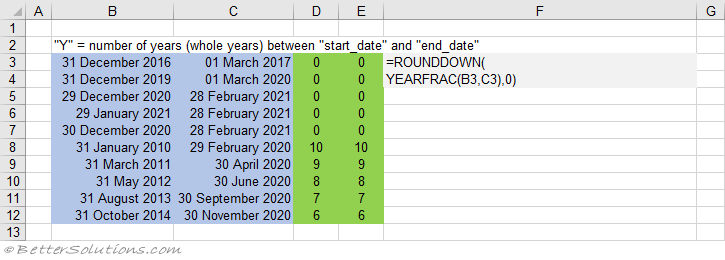### Replacement Formula - D

Column "D" uses the DATEDIF function.
Column "E" uses the formula shown.
This formula uses the function INT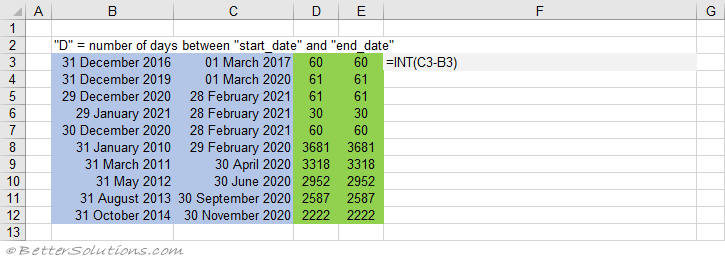### User Defined Functions

These formulas have also been converted into 6 User Defined Functions.
You can also use the DATEDIFFERENCE - User Defined Function
Column "D" uses the DATEDIF function.
Column "E" uses the DATEDIFFERENCE user defined functions.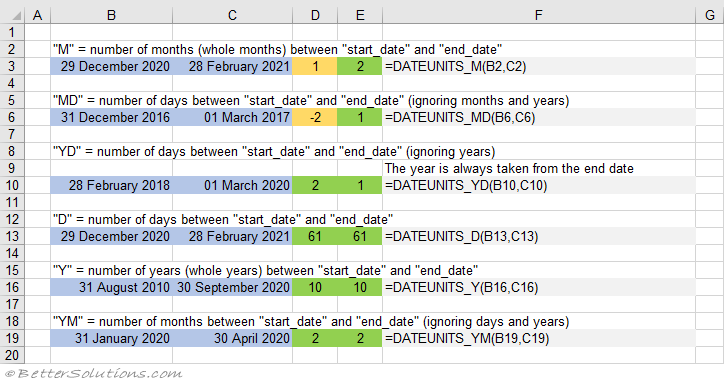### Years, Months, Days

Calculate the number of years, months, days between 2 dates.
=DATEDIF(B49,C49,"y")&" years "&DATEDIF(B49,C49,"ym")&" months "&DATEDIF(B49,C49,"md")
=IF(DATEDIF(B52,C52,"y")=0,"",DATEDIF(B52,C52,"y")&" years ")&IF(DATEDIF(B52,C52,"ym")=0,"",
=DATEDIF(B61,TODAY(),"y")&" years "&DATEDIF(B61,TODAY(),"ym")&" months "&DATEDIF(B61,TODAY(),"md")&" days"

### Important

The Excel DATEDIF function is very different to the VBA function - DATEDIFF function.
Excel thinks 1900 was a leap year.